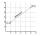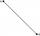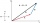# Three points

Three points A (-3;-5) B (9;-10) and C (2;k) . AB=AC
What is value of k?

Result

k1 =  7
k2 =  -17

#### Solution:Checkout calculation with our calculator of quadratic equations.Leave us a comment of example and its solution (i.e. if it is still somewhat unclear...):

Showing 0 comments:Be the first to comment!#### To solve this example are needed these knowledge from mathematics:

For Basic calculations in analytic geometry is helpful line slope calculator. From coordinates of two points in the plane it calculate slope, normal and parametric line equation(s), slope, directional angle, direction vector, the length of segment, intersections the coordinate axes etc. Pythagorean theorem is the base for the right triangle calculator.

## Next similar examples:

1. Theorem proveWe want to prove the sentense: If the natural number n is divisible by six, then n is divisible by three. From what assumption we started?
2. Vertices of a right triangleShow that the points D(2,1), E(4,0), F(5,7) are vertices of a right triangle.
3. DistanceCalculate distance between two points X[18; 19] and W[20; 3].
4. Vertices of RTShow that the points P1 (5,0), P2 (2,1) & P3 (4,7) are the vertices of a right triangle.
5. Vector - basic operationsThere are given points A [-9; -2] B [2; 16] C [16; -2] and D [12; 18] a. Determine the coordinates of the vectors u=AB v=CD s=DB b. Calculate the sum of the vectors u + v c. Calculate difference of vectors u-v d. Determine the coordinates of the vector w.
6. Right 24Right isosceles triangle has an altitude x drawn from the right angle to the hypotenuse dividing it into 2 unequal segments. The length of one segment is 5 cm. What is the area of the triangle? Thank you.
7. DistanceWha is the distance between the origin and the point (18; 22)?
8. Find the 3Find the distance and mid-point between A(1,2) and B(5,5).
9. Unit vector 2DDetermine coordinates of unit vector to vector AB if A[-6; 8], B[-18; 10].
10. ABS CNCalculate the absolute value of complex number -15-29i.
11. Triangle IRTIn isosceles right triangle ABC with right angle at vertex C is coordinates: A (-1, 2); C (-5, -2) Calculate the length of segment AB.
12. SegmentCalculate the length of the segment AB, if the coordinates of the end vertices are A[10, -4] and B[5, 5].
13. Euclid2In right triangle ABC with right angle at C is given side a=27 and height v=12. Calculate the perimeter of the triangle.
14. Vector 7Given vector OA(12,16) and vector OB(4,1). Find vector AB and vector |A|.
15. The fenceI'm building a fence. Late is rounded up in semicircle. The tops of late in the field between the columns are to copy an imaginary circle. The tip of the first and last lath in the field is a circle whose radius is unknown. The length of the circle chord i
16. TetrahedronCalculate height and volume of a regular tetrahedron whose edge has a length 18 cm.
17. Coordinates of vectorDetermine the coordinate of a vector u=CD if C(19;-7) and D(-16;-5)